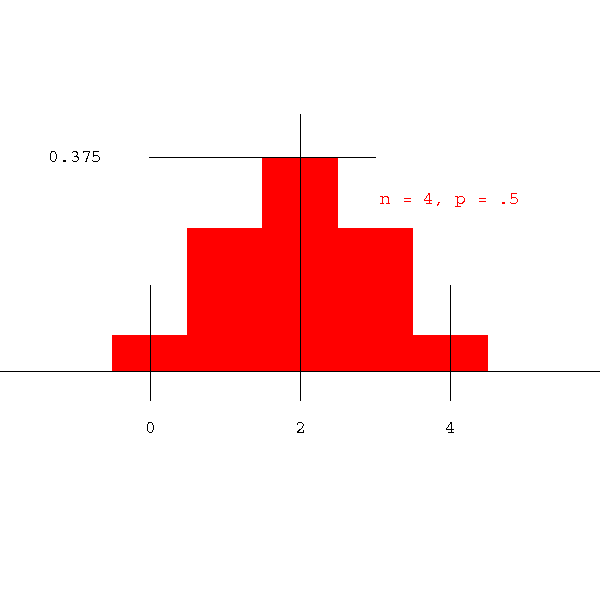### Binomial compared to normal distribution

The binomial distribution changes as n (the sample size) grows. The center of mass moves to the right, the height of the tallest column decreases and the distribution flattens out. However, the spreading that occurs is small compared to the movement of the center of mass. This means that relative to the mean of the distribution, the amount of spreading decreases.

In the display below, we correct for position and spread by adjusting the display so that center of the picture is the mean and the width is always whatever it needs to be to include 99% of the distribution. The blue curve that eventually appears is the curve to which the binomial distribution tends. It is the so-called "normal" distribution.